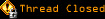# Topic awaiting preservation: The Monstruos Alliance(not what you think)!!! (Page 1 of 1)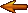sonyafterdark
Bipolar (III) Inmate

From: Bucharest, Romania, Eastern Europe
Insane since: Sep 2004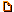posted 05-12-2005 08:21

Please help me!!! I've a system of 3 pairs of distances. Each pair consists of a spatial distance and a distance inside a plane. A 3d distance and a 2d distance. As you might have guessed, this system holds 3 texture mapping equations. I need it solved for Xm and Ym.

sqrt(sqr(Xr-X1)+sqr(Yr-Y1)+sqr(Zr-Z1))=sqrt(sqr(Xm-Xt1)+sqr(Ym-Yt1)) and
sqrt(sqr(Xr-X2)+sqr(Yr-Y2)+sqr(Zr-Z2))=sqrt(sqr(Xm-Xt2)+sqr(Ym-Yt2)) and
sqrt(sqr(Xr-X3)+sqr(Yr-Y3)+sqr(Zr-Z3))=sqrt(sqr(Xm-Xt3)+sqr(Ym-Yt3)) which

is the same with

sqr(Xr-X1)+sqr(Yr-Y1)+sqr(Zr-Z1)=sqr(Xm-Xt1)+sqr(Ym-Yt1) and
sqr(Xr-X2)+sqr(Yr-Y2)+sqr(Zr-Z2)=sqr(Xm-Xt2)+sqr(Ym-Yt2) and
sqr(Xr-X3)+sqr(Yr-Y3)+sqr(Zr-Z3)=sqr(Xm-Xt3)+sqr(Ym-Yt3)

Xr,Yr,Zr vary from case to case but whichever Pr(Xr,Yr,Zr), it is contained in the plane defined by P1(X1,Y1,Z1), P2(X2,Y2,Z2) and P3 (X3,Y3,Z3). It is also inside the triangle these form. It is the intersection between the projector ray for a given pixel and some polygon.

X1,Y1,Z1,X2,Y2,Z2,X3,Y3,Z3 also vary from case to case. These are the coordinates (in space) of the 3 triangle tips of the polygon to be texture mapped. Evidently these can be rotated and/or translated but P1, P2, P3 do not move in relation to each other. That would make the whole thing pointless, wouldn't it?

Xt1,Yt1,Xt2,Yt2,Xt3,Yt3 are unique to each polygon to be texture mapped. They are calculated when the 3D scene is loaded and never change. Not unless I morph polygons which I don't do. They are the texture coordinates assigned to each polygon tip.

So the distances between P1(X1,Y1,Z1), P2(X2,Y2,Z2) and P3(X3,Y3,Z3) don't change and are unique to each polygon.

Xm, Ym=?. Please help!!! Either solve this for me (and I'll be eternally in debt) or point me to some software that simplifies polinomials or functions.

Thanx forwardedly!!! Already posted to FreePascal.org, Chip.ro, etc.

Only posted here cos I couldn't find a math section.

F1_error

From: EN27
Insane since: Mar 2000posted 05-12-2005 16:04

Umm...wow...I'm completely lost...waaaay over my little head. But I'll have to keep an eye on this thread, as I'd love to see how it turns out.

sonyafterdark
Bipolar (III) Inmate

From: Bucharest, Romania, Eastern Europe
Insane since: Sep 2004posted 05-14-2005 08:40

My God, what a hell lot of help this is turning out to be!!!

Suho1004

From: Seoul, Korea
Insane since: Apr 2002posted 05-14-2005 09:54
quote:
sonyafterdark said:

Only posted here cos I couldn't find a math section.

That's because this isn't really a math forum...

quote:
sonyafterdark said:

My God, what a hell lot of help this is turning out to be!!!

Uh, you're welcome. We love you too.

___________________________
Suho: www.liminality.org | Cell 270 | Sig Rotator | the Fellowship of Sup

F1_error

From: EN27
Insane since: Mar 2000posted 05-14-2005 13:15

Sorry I can't help, but Suho is right, this is not a math forum, this particular area isn't even close it's "Philosophy and other Silliness".
Have you tried plugging into Google the search terms Math Forums?

poi
Paranoid (IV) Inmate

From: France
Insane since: Jun 2002posted 05-14-2005 13:34

sonyafterdark: I quickly read you post at work. I wonder if you want to retrieve the texture coordinates while still in 3D or once you've computed all the transformations and projected the thing in 2D.

Anyway I couldn't help thinking to Barycentric coordinates. If it doesn't solve your problem, it should at least lead you on the track.
Hope that helps,

ps: regarding the area of the Asylum, I think such threads would fit better in the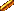OZONE or eventually in3D Modelling & Rendering.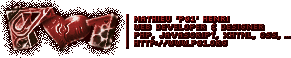sonyafterdark
Bipolar (III) Inmate

From: Bucharest, Romania, Eastern Europe
Insane since: Sep 2004posted 05-16-2005 10:43

Yes, it's nice to be loved.

I posted there too but here first and "Dan" said duplicate threads are a no-no and blocked that one. Then it disappeared altogether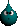.

And yes, I have tried a lot of other forums, math ones I mean - as well as Chip.ro, FreePascal.org, etc. for good measure - just as the post says in the end...

This forum really ought to have a math section too. It's a shame there isn't one.
In the meantime, as I waited for a competent and possibly useful reply (like poi's - I got antoher reply about barycentric coordinates from freepascal.org - not Suho's), I decided to compromise. It did seem unwise to try and solve the system as it was. So this morning I changed the Xts and Yts as follows:

Xt1=0;
Yt1=0;

Xt2=distance between P1(X1,Y1,Z1) and P2(X2,Y2<Z2);
Yt2=0;

Xt3=[sqr(d(P3,P1))+sqr(d(P2,P1))-sqr(d(P3,P2))]/[2*d(P2,P1)];
Yt3=[2*d(P2,P1)*sqr(d(P3,P1))-sqr(d(P3,P1))-sqr(d(P2,P1))+sqr(d(P3,P2))]/[2*d(P2,P1)];

to obtain a "FIXED" polygon surface 2D coordinate system. Note that d(P1,P2) means the distance between P1 and P2.

P1 is the origin (0,0). The line defined by P1 & P2 is the abcissa.
It is then obious that P2's ordinate coordinate is 0 whereas its abcissa coordinate is the actual distance from itself to the origin (P1).
Also obvious is that the distance from P3 to the line defined by P1 & P2 is P3's ordinate coordinate and the distance from P3's projection on the edge defined by P1 & P2 to P1 itself (the origin of the system) is its abcissa coordinate.

All this leads me to logically conclude that

Xm=[(Xr-X1)*(X2-X1)+(Yr-Y1)*(Y2-Y1)+(Zr-Z1)*(Z2-Z1)]/SQRT(SQR(X2-X1)+SQR(Y2-Y1)+SQR(Z2-Z1));
Ym=WILL HAVE TO WAIT TILL THE NEXT BREAK;

Knowing that
CurrentScreenXcoord=(Xr/Zr*ViewAngle)+HalfXResolution
we know that

Xr=(CurrentScreenXcoord-HalfXResolution)*Zr/ViewAngle. This applies to Yr as well:
Yr=(CurrentScreenYcoord-HalfYResolution)*Zr/ViewAngle. According to the 3 point definition of a plane we (should) also know:

Zr=[X1*(Y2*Z3-Y3*Z2)+X2*(Y3*Z1-Y1*Z3)+X3*(Y1*Z2-Y2*Z1)]/[X1*(Y2-Y3)+X2*(Y3-Y1)+X3*(Y1-Y2)+((CurrentScreenXcoord-HalfXResolution)*(Y1*(Z2-Z3)+Y2*(Z3-Z1)+Y3*(Z1-Z2))+(CurrentScreenYcoord-HalfYResolution)*(X1*(Z3-Z2)+X2*(Z1-Z3)+X3*(Z2-Z1))/ViewAngle].

It is plain to see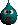that 1/Zr varies liniarly whereas Zr does not. Confusing, isn't it?
This is why a math section is needed.

This means that Xm and Ym can then PROBABLY (I'm not yet sure how) go on to be optimized for addition based liniar interpolation, just the thing needed for 3D programming. I'll find out for sure when I get there. I always find doing the math far easier than the (optimized) code for it.

But in applying this local coordinate system to the polygon I sacrifice flexibility only to gain anarchy. The effect is that the texture "hangs" from one of the polygon's edges. If that edge is crooked the texture will be too. Just like the tower of Pisa or the flag off an embassy, assuming it were rigid. It may even be upside down. Everything depends on the order the triangle tips are taken in and whether the edge the texture "hangs" from is level... The texture origin will always be in P1 (whichever one of the 3 tips P1 may be considered to be), its abcissa will always be P1-P2 (whichever one of the other 2 tips P2 may be considered to be), etc. All this means that there are 3*2=6 different ways of texturing the absolutely same polygon according to the order we count the tips in and which one we consider to be P1. This is why it is imperative one does not change the order which one stores the tips in. By sorting for example.

Cheers. 10x for the useful as well as the well-intentioned posts. Feel free to keep this goin'.